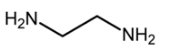Courses

# Test Level 1: Coordination Compounds

## 25 Questions MCQ Test Chemistry Class 12 | Test Level 1: Coordination Compounds

Description
This mock test of Test Level 1: Coordination Compounds for JEE helps you for every JEE entrance exam. This contains 25 Multiple Choice Questions for JEE Test Level 1: Coordination Compounds (mcq) to study with solutions a complete question bank. The solved questions answers in this Test Level 1: Coordination Compounds quiz give you a good mix of easy questions and tough questions. JEE students definitely take this Test Level 1: Coordination Compounds exercise for a better result in the exam. You can find other Test Level 1: Coordination Compounds extra questions, long questions & short questions for JEE on EduRev as well by searching above.
QUESTION: 1

Solution:

ONO- and NO2-

QUESTION: 2

### Which of the following represents chelating ligand?

Solution:QUESTION: 3

### The coordination number of Cr in  [Cr (NH3)3 (H2O)3]

Solution:

Coordination number of the central ion is equal to no. of coordination bonds formed with surrounding ions.

QUESTION: 4

Which of the following complex will give white precipitate with barium chloride solution?

Solution:

BaSO4 is precipitated when SO42- ion is present outside the coordination sphere.

QUESTION: 5

The IUPAC name [CoCl(NO2)(en)2]Cl is

Solution:

Name of ligands name first in alphabetical order followed by anme of central ion.

QUESTION: 6

The effective atomic number of Fe in Fe(CO)is

Solution:

26+2(5) = 36

QUESTION: 7

The oxidation state of Ag in tollen’s reagent is

Solution:

oxidation state of Ag in tollen’s reagent is +1.

QUESTION: 8

Which is not true about a ligand?

Solution:

Multidentate ligand always act as chelating Ligand

QUESTION: 9

In which of the following complexes, the nickel metal is in the highest oxidation state?

Solution:

In A Ni has +4 oxidation state.

QUESTION: 10

KCl.MgCl2.6H2O is a

Solution:

KCl.MgCl2.6H2O is double salt

QUESTION: 11

Which of the following can be termed as mixed complex?

Solution:

In A one and more different types of ligands are present.

QUESTION: 12

Which of the following ligand gives chelate complexes?

Solution:

Oxalate is a bidentate ligand.

QUESTION: 13

In the formation of complex entity, the central atom/ion acts as

Solution:

Central Metal ion behave as a Lewis Acid while Ligand act as a lewis Base.

QUESTION: 14

Which of the following is a negative ligand?

Solution:

C5H5a negative ligand.

QUESTION: 15

The correct IUPAC name of  Mn3(C0)12 is

Solution:

Ligands are named 1st followed by the name of central metal ion.

QUESTION: 16

Which is used in cancer therapy?

Solution:

Cis Platin (cis – [Pt(NH3)2(Cl)2] is a anti cancer drug.

QUESTION: 17

The IUPAC name of AlCl3.4(EtOH) is

Solution:

This is double salt so name is written in sequential manner.

QUESTION: 18

Sodium pentacyanonitrosylferrate(II) is also called?

Solution:

Na2[Fe(CN)5NO] is Sodium nitroprusside.

QUESTION: 19

A complex involving dsp2 hybridization has

Solution:

dsp2 is square planar geometry.

QUESTION: 20

In the complex Fe(CO)x, the value of x is

Solution:

[Fe(CO)5]

QUESTION: 21

Which among the following has square pyramidal geometry?

Solution:

QUESTION: 22

The hybridization of nickel in tetracarbonyl nickel is

Solution:

[Ni(CO)4] has tetrahedral geometry so hybridisation is sp3

QUESTION: 23

According to Werner’s theory , the primary valences of the central atom

Solution:

Primary valency is equal to no. of ligands attachted which are negatively charges.

QUESTION: 24

The EAN of Ni in [Ni(CN)4]2-- is

Solution:

EAN = 28 (Z) – 2 (oxidation number) + 2*4 (CN) = 34

Hence A

QUESTION: 25

In the complex PtCl4.3NH3 the number of ionisable chlorines is

Solution:

The complex [PtCl3(NH3)3]Cl has only one ionisable chlorine